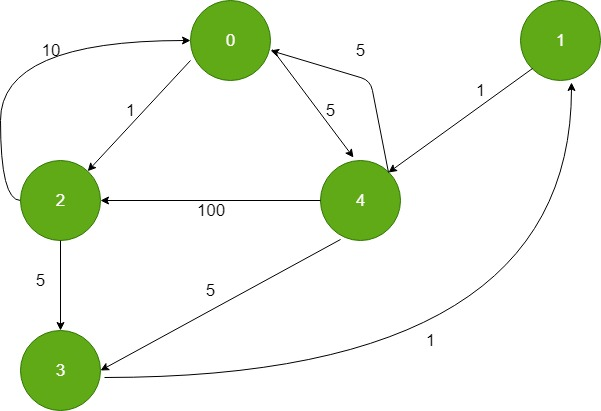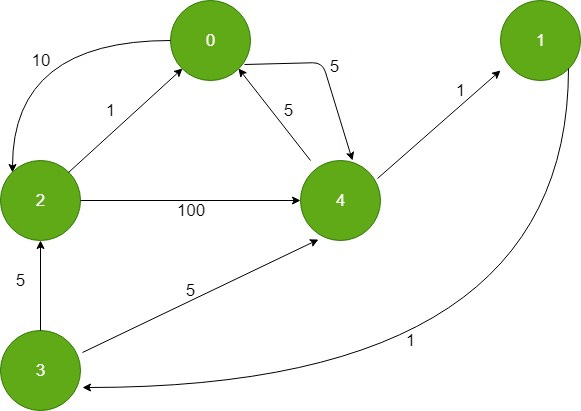GeeksforGeeks App
Open AppBrowser
Continue

# Shortest paths from all vertices to a destination

Given a Weighted Directed Graph and a destination vertex in the graph, find the shortest distance from all vertex to the destination vertex.
Input :1

Output : 0 6 10 7 5
Distance of 0 from 0: 0
Distance of 0 from 1: 1+5 = 6 (1->4->0)
Distance of 0 from 2: 10 (2->0)
Distance of 0 from 3: 1+1+5 = 7 (3->1->4->0)
Distance of 0 from 4: 5 (4->0)

Approach: The problem is similar to the Dijkstra’s problem..The idea is to use Dijkstra’s algorithm. In order to find the shortest distance from all vertex to a given destination vertex we reverse all the edges of the directed graph and use the destination vertex as the source vertex in dijkstra’s algorithm. Since all the edges are now reversed computing the shortest distance from the destination vertex to all the other vertex is similar to computing shortest distance from all vertex to a given destination vertex.
After reversing the edges the graph looks like:Now calculate the shortest distance from the destination vertex as source vertex in Dijkstra’s algorithm.
Below is the implementation of the above approach:

## CPP

 `// C++ implementation for the above approach``#include ``using` `namespace` `std;``#define INF 0x3f3f3f3f` `// iPair ==>  Integer Pair``typedef` `pair<``int``, ``int``> iPair;` `// This class represents a directed graph using``// adjacency list representation``class` `Graph {``    ``int` `V; ``// No. of vertices` `    ``// In a weighted graph, we need to store vertex``    ``// and weight pair for every edge``    ``list >* adj;` `public``:``    ``Graph(``int` `V); ``// Constructor` `    ``// function to add an reverse edge to graph``    ``void` `addEdgeRev(``int` `u, ``int` `v, ``int` `w);` `    ``// prints shortest distance from all``    ``// vertex to the given destination vertex``    ``void` `shortestPath(``int` `s);``};` `// Allocates memory for adjacency list``Graph::Graph(``int` `V)``{``    ``this``->V = V;``    ``adj = ``new` `list[V];``}` `void` `Graph::addEdgeRev(``int` `u, ``int` `v, ``int` `w)``{` `    ``adj[v].push_back(make_pair(u, w));``}` `// Prints shortest distance from all vertex to``// the given destination vertex``void` `Graph::shortestPath(``int` `dest)``{``    ``// Create a priority queue to store vertices that``    ``// are being preprocessed. This is weird syntax in C++.``    ``// Refer below link for details of this syntax``    ``// https:// www.geeksforgeeks.org/implement-min-heap-using-stl/``    ``priority_queue, greater > pq;` `    ``// Create a vector for distances and initialize all``    ``// distances as infinite (INF)``    ``vector<``int``> dist(V, INF);` `    ``// Insert destination itself in priority queue and initialize``    ``// its distance as 0.``    ``pq.push(make_pair(0, dest));``    ``dist[dest] = 0;` `    ``/* Looping till priority queue becomes empty (or all``      ``distances are not finalized) */``    ``while` `(!pq.empty()) {` `        ``// The first vertex in pair is the minimum distance``        ``// vertex, extract it from priority queue.``        ``// vertex label is stored in second of pair (it``        ``// has to be done this way to keep the vertices``        ``// sorted distance (distance must be first item``        ``// in pair)``        ``int` `u = pq.top().second;``        ``pq.pop();` `        ``// 'i' is used to get all adjacent vertices of a vertex``        ``list >::iterator i;``        ``for` `(i = adj[u].begin(); i != adj[u].end(); ++i) {` `            ``// Get vertex label and weight of current adjacent``            ``// of u.``            ``int` `v = (*i).first;``            ``int` `weight = (*i).second;` `            ``// If there is shorted path to v through u.``            ``if` `(dist[v] > dist[u] + weight) {``                ``// Updating distance of v``                ``dist[v] = dist[u] + weight;``                ``pq.push(make_pair(dist[v], v));``            ``}``        ``}``    ``}` `    ``// Print shortest distances stored in dist[]``    ``printf``(``"Destination Vertex Distance "``           ``"from all vertex\n"``);``    ``for` `(``int` `i = 0; i < V; ++i)``        ``printf``(``"%d \t\t %d\n"``, i, dist[i]);``}` `// Driver program to test methods of graph class``int` `main()``{``    ``// create the graph given in above figure``    ``int` `V = 5;``    ``Graph g(V);` `    ``// adding edges in reverse direction``    ``g.addEdgeRev(0, 2, 1);``    ``g.addEdgeRev(0, 4, 5);``    ``g.addEdgeRev(1, 4, 1);``    ``g.addEdgeRev(2, 0, 10);``    ``g.addEdgeRev(2, 3, 5);``    ``g.addEdgeRev(3, 1, 1);``    ``g.addEdgeRev(4, 0, 5);``    ``g.addEdgeRev(4, 2, 100);``    ``g.addEdgeRev(4, 3, 5);` `    ``g.shortestPath(0);` `    ``return` `0;``}`

## Java

 `/*package whatever //do not write package name here */``import` `java.io.*;``import` `java.util.*;` `// This class represents a directed graph using``// adjacency list representation``class` `Graph {``  ``int` `V;``  ``List>[] adj;``  ``Graph(``int` `V) {``    ``this``.V = V;``    ``adj = ``new` `ArrayList[V];``    ``for` `(``int` `i = ``0``; i < V; i++)``      ``adj[i] = ``new` `ArrayList>();``  ``}``  ` `  ``// function to add an reverse edge to graph``  ``void` `addEdgeRev(``int` `u, ``int` `v, ``int` `w) {``    ``adj[v].add(``new` `Pair(u, w));``  ``}``  ` `  ``// prints shortest distance from all``  ``// vertex to the given destination vertex``  ``void` `shortestPath(``int` `dest) {``    ``PriorityQueue> pq = ``new` `PriorityQueue<>(``new` `Comparator>() {``      ``public` `int` `compare(Pair p1, Pair p2) {``        ``return` `p1.getKey() - p2.getKey();``      ``}``    ``});` `    ``int``[] dist = ``new` `int``[V];``    ``Arrays.fill(dist, Integer.MAX_VALUE);` `    ``pq.add(``new` `Pair(``0``, dest));``    ``dist[dest] = ``0``;``    ` `    ``// The first vertex in pair is the minimum distance``    ``// vertex, extract it from priority queue.``    ``// vertex label is stored in second of pair (it``    ``// has to be done this way to keep the vertices``    ``// sorted distance (distance must be first item``    ``// in pair)``    ``while` `(!pq.isEmpty()) {``      ``int` `u = pq.peek().getValue();``      ``pq.poll();` `      ``for` `(Pair pair : adj[u]) {``        ``int` `v = pair.getKey();``        ``int` `weight = pair.getValue();` `        ``if` `(dist[v] > dist[u] + weight) {``          ``dist[v] = dist[u] + weight;``          ``pq.add(``new` `Pair(dist[v], v));``        ``}``      ``}``    ``}` `    ``System.out.println(``"Destination Vertex Distance from all vertex"``);``    ``for` `(``int` `i = ``0``; i < V; i++)``      ``System.out.println(i + ``" \t\t "` `+ dist[i]);``  ``}``  ` `  ``// Driver code``  ``public` `static` `void` `main(String[] args) {``    ``Graph g = ``new` `Graph(``5``);` `    ``g.addEdgeRev(``0``, ``2``, ``1``);``    ``g.addEdgeRev(``0``, ``4``, ``5``);``    ``g.addEdgeRev(``1``, ``4``, ``1``);``    ``g.addEdgeRev(``2``, ``0``, ``10``);``    ``g.addEdgeRev(``2``, ``3``, ``5``);``    ``g.addEdgeRev(``3``, ``1``, ``1``);``    ``g.addEdgeRev(``4``, ``0``, ``5``);``    ``g.addEdgeRev(``4``, ``2``, ``100``);``    ``g.addEdgeRev(``4``, ``3``, ``5``);` `    ``g.shortestPath(``0``);``  ``}``}` `class` `Pair {``  ``K key;``  ``V value;``  ``Pair(K key, V value) {``    ``this``.key = key;``    ``this``.value = value;``  ``}` `  ``K getKey() {``    ``return` `key;``  ``}` `  ``V getValue() {``    ``return` `value;``  ``}``}` `// This code is contributed by lokeshpotta20.`

## Python3

 `from` `queue ``import` `PriorityQueue``INF ``=` `int``(``0x3f3f3f3f``)` `# This class represents a directed graph using``# adjacency list representation``class` `Graph:``    ``def` `__init__(``self``, V: ``int``) ``-``> ``None``:` `        ``self``.V ``=` `V``        ``# No. of vertices` `        ``# In a weighted graph, we need to store vertex``        ``# and weight pair for every edge``        ``self``.adj ``=` `[[] ``for` `_ ``in` `range``(V)]``    ``def` `addEdgeRev(``self``, u: ``int``, v: ``int``, w: ``int``) ``-``> ``None``:``        ``self``.adj[v].append((u, w))` `    ``# Prints shortest distance from all vertex to``    ``# the given destination vertex``    ``def` `shortestPath(``self``, dest: ``int``) ``-``> ``None``:` `        ``# Create a priority queue to store vertices that``        ``# are being preprocessed. This is weird syntax in C++.``        ``# Refer below link for details of this syntax``        ``# https:# www.geeksforgeeks.org/implement-min-heap-using-stl/``        ``pq ``=` `PriorityQueue()` `        ``# Create a vector for distances and initialize all``        ``# distances as infinite (INF)``        ``dist ``=` `[INF ``for` `_ ``in` `range``(V)]` `        ``# Insert destination itself in priority queue and initialize``        ``# its distance as 0.``        ``pq.put((``0``, dest))``        ``dist[dest] ``=` `0` `        ``# Looping till priority queue becomes empty (or all``        ``# distances are not finalized) */``        ``while` `not` `pq.empty():` `            ``# The first vertex in pair is the minimum distance``            ``# vertex, extract it from priority queue.``            ``# vertex label is stored in second of pair (it``            ``# has to be done this way to keep the vertices``            ``# sorted distance (distance must be first item``            ``# in pair)``            ``u ``=` `pq.get()[``1``]` `            ``# 'i' is used to get all adjacent vertices of a vertex``            ``for` `i ``in` `self``.adj[u]:` `                ``# Get vertex label and weight of current adjacent``                ``# of u.``                ``v ``=` `i[``0``]``                ``weight ``=` `i[``1``]` `                ``# If there is shorted path to v through u.``                ``if` `(dist[v] > dist[u] ``+` `weight):``                    ` `                    ``# Updating distance of v``                    ``dist[v] ``=` `dist[u] ``+` `weight``                    ``pq.put((dist[v], v))` `        ``# Print shortest distances stored in dist[]``        ``print``(``"Destination Vertex Distance from all vertex"``)``        ``for` `i ``in` `range``(V):``            ``print``(``"{} \t\t {}"``.``format``(i, dist[i]))` `# Driver code``if` `__name__ ``=``=` `"__main__"``:` `    ``# create the graph given in above figure``    ``V ``=` `5``    ``g ``=` `Graph(V)` `    ``# adding edges in reverse direction``    ``g.addEdgeRev(``0``, ``2``, ``1``)``    ``g.addEdgeRev(``0``, ``4``, ``5``)``    ``g.addEdgeRev(``1``, ``4``, ``1``)``    ``g.addEdgeRev(``2``, ``0``, ``10``)``    ``g.addEdgeRev(``2``, ``3``, ``5``)``    ``g.addEdgeRev(``3``, ``1``, ``1``)``    ``g.addEdgeRev(``4``, ``0``, ``5``)``    ``g.addEdgeRev(``4``, ``2``, ``100``)``    ``g.addEdgeRev(``4``, ``3``, ``5``)` `    ``g.shortestPath(``0``)` `# This code is contributed by sanjeev2552`

## Javascript

 `class PriorityQueue {``  ``constructor() {``    ``this``.heap = [];``  ``}` `  ``push(val) {``    ``this``.heap.push(val);``    ``this``.bubbleUp(``this``.heap.length - 1);``  ``}` `  ``pop() {``    ``const poppedVal = ``this``.heap;``    ``const bottom = ``this``.heap.length - 1;``    ``if` `(bottom > 0) {``      ``this``.swap(0, bottom);``    ``}``    ``this``.heap.pop();``    ``this``.sinkDown(0);``    ``return` `poppedVal;``  ``}` `  ``bubbleUp(idx) {``    ``const element = ``this``.heap[idx];``    ``while` `(idx > 0) {``      ``const parentIdx = Math.floor((idx + 1) / 2) - 1;``      ``const parent = ``this``.heap[parentIdx];``      ``if` `(element < parent) {``        ``this``.swap(idx, parentIdx);``        ``idx = parentIdx;``      ``} ``else` `{``        ``break``;``      ``}``    ``}``  ``}` `  ``sinkDown(idx) {``    ``const length = ``this``.heap.length;``    ``const element = ``this``.heap[idx];``    ``while` `(``true``) {``      ``const leftChildIdx = 2 * (idx + 1) - 1;``      ``const rightChildIdx = 2 * (idx + 1);``      ``let leftChild, rightChild;``      ``let swapIdx = ``null``;` `      ``if` `(leftChildIdx < length) {``        ``leftChild = ``this``.heap[leftChildIdx];``        ``if` `(leftChild < element) {``          ``swapIdx = leftChildIdx;``        ``}``      ``}` `      ``if` `(rightChildIdx < length) {``        ``rightChild = ``this``.heap[rightChildIdx];``        ``if` `(``          ``(swapIdx === ``null` `&& rightChild < element) ||``          ``(swapIdx !== ``null` `&& rightChild < leftChild)``        ``) {``          ``swapIdx = rightChildIdx;``        ``}``      ``}` `      ``if` `(swapIdx === ``null``) {``        ``break``;``      ``}` `      ``this``.swap(idx, swapIdx);``      ``idx = swapIdx;``    ``}``  ``}` `  ``swap(idx1, idx2) {``    ``[``this``.heap[idx1], ``this``.heap[idx2]] = [``this``.heap[idx2], ``this``.heap[idx1]];``  ``}` `  ``get length() {``    ``return` `this``.heap.length;``  ``}` `  ``get empty() {``    ``return` `this``.heap.length === 0;``  ``}``}` `const INF = 0x3f3f3f3f;``// This class represents a directed graph using``// adjacency list representation``class Graph {``  ``constructor(V) {``    ``this``.V = V;``    ` `    ``this``.adj = Array.from({ length: V }, () => []);``  ``}``  ` `  ``// function to add an reverse edge to graph``  ``addEdgeRev(u, v, w) {``    ``this``.adj[v].push([u, w]);``  ``}``  ``// prints shortest distance from all``  ``// vertex to the given destination vertex``  ``shortestPath(dest) {``    ``const pq = ``new` `PriorityQueue();``    ``const dist = Array.from({ length: ``this``.V }, () => INF);``    ``pq.push([0, dest]);``    ``dist[dest] = 0;``        ` `    ``// The first vertex in pair is the minimum distance``    ``// vertex, extract it from priority queue.``    ``// vertex label is stored in second of pair (it``    ``// has to be done this way to keep the vertices``    ``// sorted distance (distance must be first item``    ``// in pair)``    ``while` `(!pq.empty) {``      ``const [uDist, u] = pq.pop();``      ``for` `(const [v, weight] of ``this``.adj[u]) {``        ``if` `(dist[v] > dist[u] + weight) {``          ``dist[v] = dist[u] + weight;``          ``pq.push([dist[v], v]);``        ``}``      ``}``    ``}``    ``console.log(``"Destination Vertex Distance from all vertex"``);``    ``for` `(let i = 0; i < ``this``.V; i++) {``      ``document.write(`\${i}           \${dist[i]}`);``    ``}``  ``}``}``//Driver code``const V = 5;``const g = ``new` `Graph(V);``g.addEdgeRev(0, 2, 1);``g.addEdgeRev(0, 4, 5)``    ``g.addEdgeRev(1, 4, 1)``    ``g.addEdgeRev(2, 0, 10)``    ``g.addEdgeRev(2, 3, 5)``    ``g.addEdgeRev(3, 1, 1)``    ``g.addEdgeRev(4, 0, 5)``    ``g.addEdgeRev(4, 2, 100)``    ``g.addEdgeRev(4, 3, 5)` `    ``g.shortestPath(0)`

## C#

 `using` `System;``using` `System.Collections.Generic;` `// This class represents a directed graph using``// adjacency list representation``class` `Graph {``    ``private` `int` `V;``    ``private` `List >[] adj;` `    ``public` `Graph(``int` `V)``    ``{``        ``this``.V = V;``        ``adj = ``new` `List >[ V ];``        ``for` `(``int` `i = 0; i < V; i++) {``            ``adj[i] = ``new` `List >();``        ``}``    ``}``    ``// function to add an reverse edge to graph``    ``// prints shortest distance from all``    ``// vertex to the given destination vertex``    ``void` `addEdgeRev(``int` `u, ``int` `v, ``int` `w)``    ``{``        ``adj[v].Add(``new` `KeyValuePair<``int``, ``int``>(u, w));``    ``}` `    ``// prints shortest distance from all``    ``// vertex to the given destination vertex``    ``public` `void` `shortestPath(``int` `dest)``    ``{``        ``SortedSet > pq``            ``= ``new` `SortedSet >(``                ``Comparer >.Create(``                    ``(a, b) = > a.Key.CompareTo(b.Key)));` `        ``int``[] dist = ``new` `int``[V];``        ``Array.Fill(dist, ``int``.MaxValue);` `        ``pq.Add(``new` `KeyValuePair<``int``, ``int``>(0, dest));``        ``dist[dest] = 0;``        ``// The first vertex in pair is the minimum distance``        ``// vertex, extract it from priority queue.``        ``// vertex label is stored in second of pair (it``        ``// has to be done this way to keep the vertices``        ``// sorted distance (distance must be first item``        ``// in pair)``        ``while` `(pq.Count > 0) {``            ``int` `u = pq.Min.Value;``            ``pq.Remove(pq.Min);` `            ``foreach``(KeyValuePair<``int``, ``int``> pair ``in` `adj[u])``            ``{``                ``int` `v = pair.Key;``                ``int` `weight = pair.Value;` `                ``if` `(dist[v] > dist[u] + weight) {``                    ``dist[v] = dist[u] + weight;``                    ``pq.Add(``new` `KeyValuePair<``int``, ``int``>(``                        ``dist[v], v));``                ``}``            ``}``        ``}` `        ``Console.WriteLine(``            ``"Destination Vertex Distance from all vertex"``);``        ``for` `(``int` `i = 0; i < V; i++) {``            ``Console.WriteLine(i + ``" \t\t "` `+ dist[i]);``        ``}``    ``}``    ``// Driver code``    ``public` `static` `void` `Main(``string``[] args)``    ``{``        ``Graph g = ``new` `Graph(5);` `        ``g.addEdgeRev(0, 2, 1);``        ``g.addEdgeRev(0, 4, 5);``        ``g.addEdgeRev(1, 4, 1);``        ``g.addEdgeRev(2, 0, 10);``        ``g.addEdgeRev(2, 3, 5);``        ``g.addEdgeRev(3, 1, 1);``        ``g.addEdgeRev(4, 0, 5);``        ``g.addEdgeRev(4, 2, 100);``        ``g.addEdgeRev(4, 3, 5);` `        ``g.shortestPath(0);``    ``}``}`

Output:

```Destination Vertex Distance from all vertex
0                0
1                6
2                10
3                7
4                5         ```

My Personal Notes arrow_drop_up